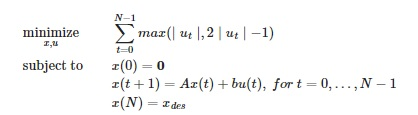# How to write state space form as constraints in cvx in matlab

Hello, I have this problem written in python .
I want to convert it to matlab just the part for state space and the initial and final conditions
N = 30
A = [[-1. 0.4 0.8]
[ 1. 0. 0. ]
[ 0. 1. 0. ]]
b = [[ 1. ]
[ 0. ]
[ 0.3]]
Xdes = [ 7. 2. -6.]

X = Variable(n, N+1)
u = Variable(1, N)
obj = Minimize(sum_entries(max_elemwise(abs(u), 2abs(u)-1)))
constr = [X[:, 0] == 0, X[:, 1:] == A
X[:,:N] + b*u, X[:, -1] == xdes]
prob = Problem(obj, constr)Start by reading the CVX Users’ Guide.

You can use `==` for an equality constraint.

``````X(:,1) == 0
X(:,N+1) == Xdes'
``````

I’ll let you figure out the rest.

Thanks for your help, but I am wondering how should I put the state space as a constraint itself…shall I put it inisde a loop and the whole CVX inside another big loop or what?

Like this :
For i=1:N+1

cvx_begin
variable X(n, N+1);
variable u(1,N);
Obj=0

For j=1:N-1
Obj=obj+max(…,…)
end
minimize (obj);

Subject to

X(:,1) == 0
X(:,N+1) == Xdes’
For k=1:N+1
X(k+1)==A*X(k)+bu(k)
end

cvx_end
end
Or is this wrong?

There should not be a for loop around (outside) cvx_begin … cvx_end, because you only want to solve one optimization problem. All CVX statements about the optimization problem you are solving needs to be inside cvx_begin … cvx_end Only use a for loop around cvx_begin … cvx_end if you are solving more than one optimization problem (for instance, with different input data), or where the results of one optimization problem become input data for the next optimization problem.

Your last for loop should have for k = 1:N-1. As it is now, you not only go past the end of the array, you are setting up a conflicting equality constraint for X(N+1), given you also have a constraint just on X(N+1). The image you show does seem to have that conflict; so I will leave that to you to sort out; I suspect that is carelessness (or typo) on that part of the paper or book authors.

If you look at the help for `max`, you will see that you can vectorze the calculation and avoid the for loop, which saves time in CVX’s processing. But using a for loop is not “wrong”.

Thank you for helping me out.
I solved the problem now by imposing
the constraints as

X(:,1) == zeros(3,1);
X(:,N)==Xdes’;
for k=1:N-1
X(:,k+1)== AX(:,k)+bu(k);
end

Shouldn’t that be
`X(:,N+1)==Xdes’`
?

Your code imposes two different constraints on `X(:,N)`

I think you are right after I went over it again regarding the last piece of code I sent . But, after viewing ‘X’ in Matlab, I realized that the 30th column of X is set to the desired value as I set it in X(:,N)==Xdes’; and the 31st column is [0 0 0]’.

It looks like CVX does not overwrite the constraints in the same way of overwriting a variable in Matlab.

In other words, although what you mentioned is totally true, it seems that CVX, when the 30th column of constraints was called, didn’t change the already-set value of the (X(:,N)==Xdes’; ) somehow inside the for-loop.

here is a print of the columns of X ( in red : the 30th column)

I don’t know exactly how CVX works, but I recommend using “correct” code.

In the following case, CVX imposes two (conflicting) constraints.

``````cvx_begin
variable x
x==1
x==2
cvx_end
``````

Trivial infeasibilities detected; solution determined analytically.
Status: Infeasible
Optimal value (cvx_optval): +Inf

++++++++++++++++++++++++++++++

Assignments ( `=` ) are always overwritten (updated),

Will the CVX show a warning if I am reassinging a constraint?

I showed the entirety of the output in my example. There was no warning.